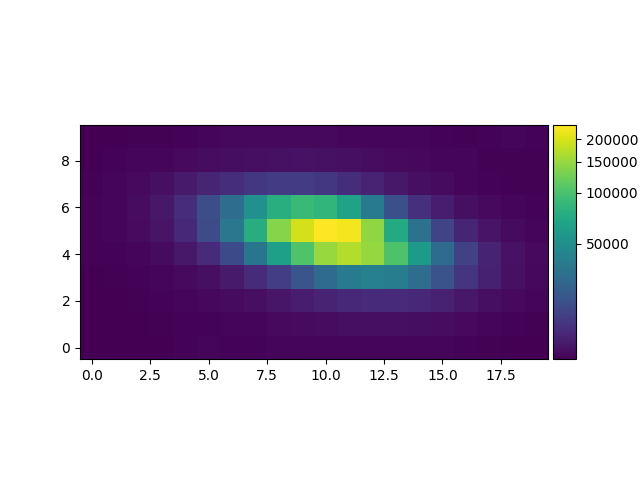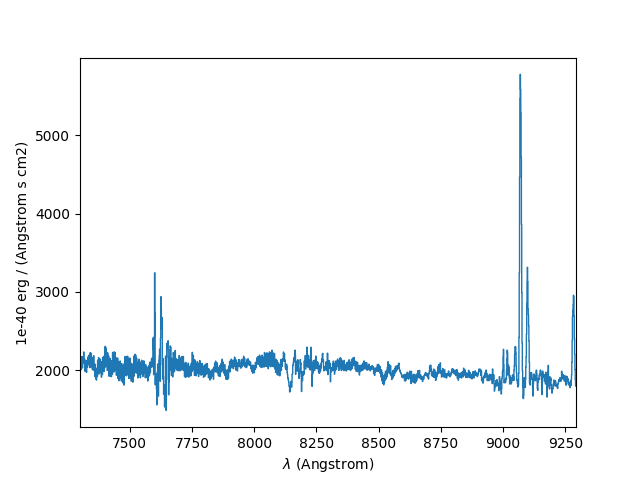# Getting Started¶

## Importing MPDAF¶

MPDAF is divided into sub-packages, each of which is composed of several classes. The following example shows how to import the Cube and PixTable classes:

In : from mpdaf.obj import Cube

In : from mpdaf.drs import PixTable


All of the examples in the MPDAF web pages are shown being typed into an interactive IPython shell. This shell is the origin of the prompts like In : in the above example. The examples can also be entered in other shells, such as the native Python shell.

MUSE datacubes are generally loaded from FITS files. In these files the fluxes and variances are stored in separate FITS extensions. For example:

# data and variance arrays are read from DATA and STAT extensions of the file
In : cube = Cube('obj/CUBE.fits')

In : cube.info()
[INFO] 1595 x 10 x 20 Cube (obj/CUBE.fits)
[INFO] .data(1595 x 10 x 20) (1e-40 erg / (Angstrom cm2 s)), .var(1595 x 10 x 20)
[INFO] center:(-30:00:00.4494,01:20:00.4376) size:(2.000",4.000") step:(0.200",0.200") rot:-0.0 deg frame:FK5
[INFO] wavelength: min:7300.00 max:9292.50 step:1.25 Angstrom


The listed dimensions of the cube, 1595 x 10 x 20, indicate that the cube has 1595 spectral pixels and 10 x 20 spatial pixels. The order in which these dimensions are listed, follows the indexing conventions used by Python to handle 3D arrays (see Spectrum, Image and Cube format for more information).

Let’s compute the reconstructed white-light image and display it. The white-light image is obtained by summing each spatial pixel of the cube along the wavelength axis. This converts the 3D cube into a 2D image.

In : ima = cube.sum(axis=0)

In : type(ima)
Out: mpdaf.obj.image.Image

In : plt.figure()
Out: <Figure size 640x480 with 0 Axes>

In : ima.plot(scale='arcsinh', colorbar='v')
Out: <matplotlib.image.AxesImage at 0x7fdd1c8538d0>Let’s now compute the overall spectrum of the cube by taking the cube and summing along the X and Y axes of the image plane. This yields the total flux per spectral pixel.

In : sp = cube.sum(axis=(1,2))

In : type(sp)
Out: mpdaf.obj.spectrum.Spectrum

In : plt.figure()
Out: <Figure size 640x480 with 0 Axes>

In : sp.plot()## Logging¶

When imported, MPDAF initialize a logger by default. This logger uses the logging module, and log messages to stderr, for instance for the .info() methods. See Logging (mpdaf.log) for more details.

Because different sub-packages have very different functionality, further suggestions for getting started are provided in the online documentation of these sub-packages. For example, click on Cube object, Image object, or Spectrum object for help with the 3 main classes of the mpdaf.obj package.

Alternatively, if you use the IPython interactive python shell, then you can look at the docstrings of classes, objects and functions by following them with the magic ? of IPython. Examples of this are shown below. A more general way to see these docstrings, which works in all Python shells, is to use the built-in help() function:

In : Cube.median?
Signature: Cube.median(self, axis=None)
Docstring:
Return the median over a given axis.

Beware that if the pixels of the cube have associated variances, these
are discarded by this function, because there is no way to estimate the
effects of a median on the variance.

Parameters
----------
axis : int or tuple of int, optional
The axis or axes along which a median is performed.

The default (axis = None) performs a median over all the
dimensions of the cube and returns a float.

axis = 0 performs a median over the wavelength dimension and
returns an image.

axis = (1,2) performs a median over the (X,Y) axes and
returns a spectrum.
Type:      function

In : help(ima.plot)
Help on method plot in module mpdaf.obj.image:

plot(title=None, scale='linear', vmin=None, vmax=None, zscale=False, colorbar=None, var=False, show_xlabel=False, show_ylabel=False, ax=None, unit=Unit("deg"), use_wcs=False, **kwargs) method of mpdaf.obj.image.Image instance
Plot the image with axes labeled in pixels.

If either axis has just one pixel, plot a line instead of an image.

Colors are assigned to each pixel value as follows. First each
pixel value, pv, is normalized over the range vmin to vmax,
to have a value nv, that goes from 0 to 1, as follows::

nv = (pv - vmin) / (vmax - vmin)

This value is then mapped to another number between 0 and 1 which
determines a position along the colorbar, and thus the color to give
the displayed pixel. The mapping from normalized values to colorbar
position, color, can be chosen using the scale argument, from the
following options:

- 'linear': color = nv
- 'log': color = log(1000 * nv + 1) / log(1000 + 1)
- 'sqrt': color = sqrt(nv)
- 'arcsinh': color = arcsinh(10*nv) / arcsinh(10.0)

A colorbar can optionally be drawn. If the colorbar argument is given
the value 'h', then a colorbar is drawn horizontally, above the plot.
If it is 'v', the colorbar is drawn vertically, to the right of the
plot.

By default the image is displayed in its own plot. Alternatively
to make it a subplot of a larger figure, a suitable
matplotlib.axes.Axes object can be passed via the ax argument.
Note that unless matplotlib interative mode has previously been enabled
by calling matplotlib.pyplot.ion(), the plot window will not appear
until the next time that matplotlib.pyplot.show() is called. So to
arrange that a new window appears as soon as Image.plot() is
called, do the following before the first call to Image.plot()::

import matplotlib.pyplot as plt
plt.ion()

Parameters
----------
title : str
An optional title for the figure (None by default).
scale : 'linear' | 'log' | 'sqrt' | 'arcsinh'
The stretch function to use mapping pixel values to
colors (The default is 'linear'). The pixel values are
first normalized to range from 0 for values <= vmin,
to 1 for values >= vmax, then the stretch algorithm maps
these normalized values, nv, to a position p from 0 to 1
along the colorbar, as follows:
linear:  p = nv
log:     p = log(1000 * nv + 1) / log(1000 + 1)
sqrt:    p = sqrt(nv)
arcsinh: p = arcsinh(10*nv) / arcsinh(10.0)
vmin : float
Pixels that have values <= vmin are given the color
at the dark end of the color bar. Pixel values between
vmin and vmax are given colors along the colorbar according
to the mapping algorithm specified by the scale argument.
vmax : float
Pixels that have values >= vmax are given the color
at the bright end of the color bar. If None, vmax is
set to the maximum pixel value in the image.
zscale : bool
If True, vmin and vmax are automatically computed
using the IRAF zscale algorithm.
colorbar : str
If 'h', a horizontal colorbar is drawn above the image.
If 'v', a vertical colorbar is drawn to the right of the image.
If None (the default), no colorbar is drawn.
var : bool
If true variance array is shown in place of data array
ax : matplotlib.axes.Axes
An optional Axes instance in which to draw the image,
or None to have one created using matplotlib.pyplot.gca().
unit : astropy.units.Unit
The units to use for displaying world coordinates
(degrees by default). In the interactive plot, when
the mouse pointer is over a pixel in the image the
coordinates of the pixel are shown using these units,
along with the pixel value.
use_wcs : bool
If True, use astropy.visualization.wcsaxes to get axes
with world coordinates.
kwargs : matplotlib.artist.Artist
Optional extra keyword/value arguments to be passed to
the ax.imshow() function.

Returns
-------
out : matplotlib AxesImage